# 聚类实战：一维数组数据聚类钱魏Way · · 8 次浏览

• 需求内容：分析订单的价格分布
• 常见方案：按照100为梯度，分析不同价格区间的订单量
• 存在缺陷：现实生活中，定价存在一些自然的价格分隔，如果按照步距划分可能存在一些偏差，比如airbnb的价格筛选显示出的房价分布：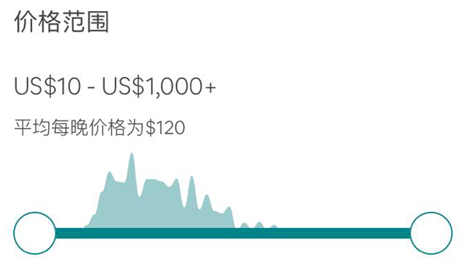## 方案一：采用K-Means对一维数据聚类

Python代码如下：

from sklearn.cluster import KMeans
import numpy as np
x = np.random.random(10000)
y = x.reshape(-1,1)
km = KMeans()
km.fit(y)
km.cluster_centers_


## 方案二：采用一维聚类方法Jenks Natural Breaks

Jenks Natural Breaks（自然断点分类）。一般来说，分类的原则就是差不多的放在一起，分成若干类。统计上可以用方差来衡量，通过计算每类的方差，再计算这些方差之和，用方差和的大小来比较分类的好坏。因而需要计算各种分类的方差和，其值最小的就是最优的分类结果（但并不唯一）。这也是自然断点分类法的原理。另外，当你去看数据的分布时，可以比较明显的发现断裂之处，这些断裂之处和Jenks Natural Breaks方法算出来也是一致的。因而这种分类法很“自然”。

Jenks Natural Breaks和K Means在一维数据时，完全等价。它们的目标函数一样，但是算法的步骤不完全相同。K Means是先设定好K个初始随机点。而Jenks Breaks则是用遍历的方法，一个点一个点地移动，直到达到最小值。

Natural Breaks算法又有两种：

• Jenks-Caspall algorithm(1971)，是Jenks和Caspall发明的算法。原理就如前所述，实现的时候要将每种分类情况都计算一遍，找到方差和最小的那一种，计算量极大。n个数分成k类，就要从n-1个数中找k-1个组合，这个数目是很惊人的。数据量较大时，如果分类又多，以当时的计算机水平根本不能穷举各种可能性。
• Fisher-Jenks algorithm(1977)，Fisher(1958)发明了一种算法提高计算效率，不需要进行穷举。Jenks将这种方法引入到数据分类中。但后来者几乎只知道Jenks而不知Fisher了。

$$SDCM=\sum_{i=1}^{k} \frac{1}{N_{i}} \sum_{i=1}^{N_{i}}(z_{i j}-\bar{z}_{j})^{2}$$

$$SDAM=\frac{1}{N} \sum_{i=1}^{N}\left(z_{i}-\bar{z}\right)^{2}$$

$$GVF=1-\frac{SDMC}{SDAM}$$

GVF用于判定不同分类数的分类效果好坏。以k和GVF做图可得：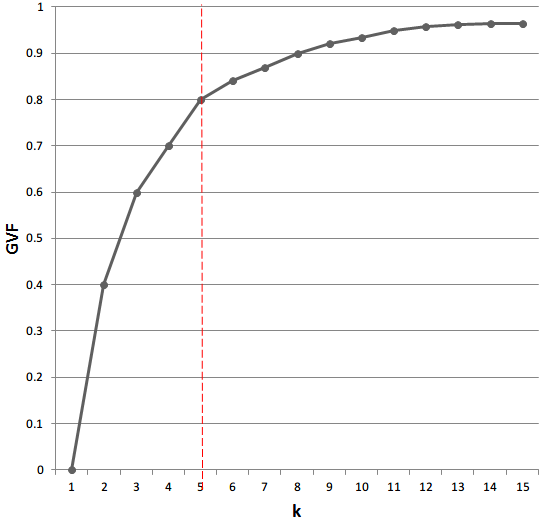from jenkspy import jenks_breaks
import numpy as np

def goodness_of_variance_fit(array, classes):
# get the break points
classes = jenks_breaks(array, classes)

# do the actual classification
classified = np.array([classify(i, classes) for i in array])

# max value of zones
maxz = max(classified)

# nested list of zone indices
zone_indices = [[idx for idx, val in enumerate(classified) if zone + 1 == val] for zone in range(maxz)]

# sum of squared deviations from array mean
sdam = np.sum((array - array.mean()) ** 2)

# sorted polygon stats
array_sort = [np.array([array[index] for index in zone]) for zone in zone_indices]

# sum of squared deviations of class means
sdcm = sum([np.sum((classified - classified.mean()) ** 2) for classified in array_sort])

# goodness of variance fit
gvf = (sdam - sdcm) / sdam

return gvf

def classify(value, breaks):
for i in range(1, len(breaks)):
if value < breaks[i]:
return i
return len(breaks) - 1

if __name__ == '__main__':
gvf = 0.0
nclasses = 2
array = np.random.random(10000)
while gvf < .8:
gvf = goodness_of_variance_fit(array, nclasses)
print(nclasses, gvf)
nclasses += 1


## 方案三：核密度估计Kernel Density Estimation

import numpy as np
from scipy.signal import argrelextrema
import matplotlib.pyplot as plt
from sklearn.neighbors.kde import KernelDensity

a = np.array([10, 11, 9, 23, 21, 11, 45, 20, 11, 12]).reshape(-1, 1)
kde = KernelDensity(kernel='gaussian', bandwidth=3).fit(a)
s = np.linspace(0, 50)
e = kde.score_samples(s.reshape(-1, 1))
plt.plot(s, e)
plt.show()

mi, ma = argrelextrema(e, np.less), argrelextrema(e, np.greater)
print("Minima:", s[mi])
print("Maxima:", s[ma])
print(a[a < mi], a[(a >= mi) * (a <= mi)], a[a >= mi])

plt.plot(s[:mi + 1], e[:mi + 1], 'r',
s[mi:mi + 1], e[mi:mi + 1], 'g',
s[mi:], e[mi:], 'b',
s[ma], e[ma], 'go',
s[mi], e[mi], 'ro')
plt.show()

Minima: [17.34693878 33.67346939]
Maxima: [10.20408163 21.42857143 44.89795918]
[10 11  9 11 11 12] [23 21 20]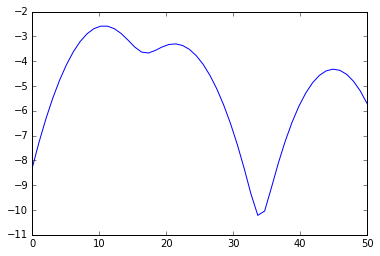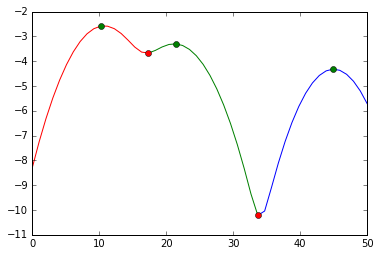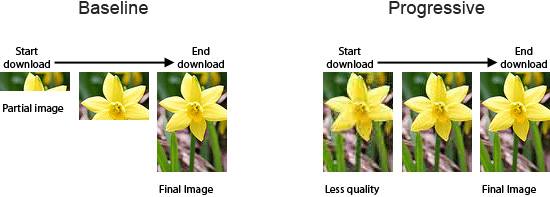##### 使用渐进式JPEG来提升用户体验##### 网站开发图片格式对比与选择